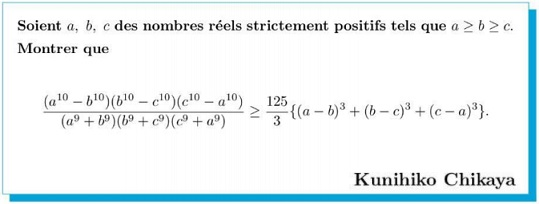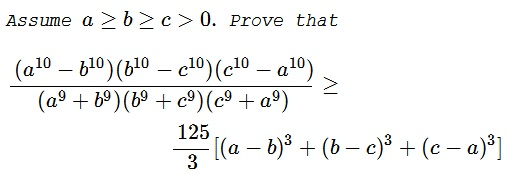# Kunihiko Chikaya's Inequality

### Source### Problem### Solution 1

Under the constraints, the inequality is equivalent to

$\displaystyle \small{\frac{(a^{10}-b^{10})(b^{10}-c^{10})(a^{10}-c^{10})}{(a^{9}+b^{9})(b^{9}+c^{9})(c^{9}+a^{9})}\ge\frac{125}{3}[(a-c)^3-(a-b)^3-(b-c)^3]}.$

But

$\displaystyle (a-c)^3-(a-b)^3-(b-c)^3=3(a-b)(b-c)(a-c).$

Hence, suffice it to show that

$\displaystyle \frac{(a^{10}-b^{10})(b^{10}-c^{10})(a^{10}-c^{10})}{(a^{9}+b^{9})(b^{9}+c^{9})(c^{9}+a^{9})}\ge 125(a-b)(b-c)(a-c).$

By the AM-GM inequality, employed repeatedly, or by Muirhead's inequality, employed just once,

$\displaystyle \frac{u^{10}-v^{10}}{u-v}\le 5(u^9+v^9).$

Substituting $a,b,c$ in pairs and taking the product proves the required inequality.

### Solution 2

The $RHS$ simplifies to $125(a-b)(b-c)(c-a)$. If any two variables are equal, the inequality is trivially satisfied. Thus, we assume that no two variables are equal.

The constraint implies that both $LHS$ and $RHS$ are non-positive. Thus, we can negate both sides and flip the direction of the inequality. The inequality can then be written as

\begin{align} &&\left[5(a-b)(a^9+b^9)\right]\left[5(b-c)(b^9+c^9)\right]\left[5(a-c)(a^9+c^9)\right] \\ &&\geq (a^{10}-b^{10}) (b^{10}-c^{10}) (a^{10}-c^{10}). \end{align}

If we prove that for $x>y$,

$5(x-y)(x^9+y^9)\geq (x^{10}-y^{10}),$

we are done. Dropping the common positive factor $(x-y)$ the inequality becomes

\displaystyle \begin{align} &5(x^9+y^9)-\sum_{i=0}^{9}x^iy^{9-i}\geq 0 \\ &[(x^9+y^9)-(x^8y+y^8x)]+[(x^9+y^9)-(x^7y^2+y^7x^2)]+ \\ &[(x^9+y^9)-(x^6y^3+y^6x^3)]+ [(x^9+y^9)-(x^5y^4+y^5x^4)]\geq 0. \end{align}

Each box bracket is $\geq 0$ from Muirhead and the inequality follows.

### Solution 3

The $RHS$ simplifies to $125(a-b)(b-c)(c-a)$. If any two variables are equal, the inequality is trivially satisfied. Thus, we assume that no two variables are equal.

The constraint implies that both $LHS$ and $RHS$ are non-positive. Thus, we can negate both sides and flip the direction of the inequality. The inequality can then be written as

\begin{align} &&\left[5(a-b)(a^9+b^9)\right]\left[5(b-c)(b^9+c^9)\right]\left[5(a-c)(a^9+c^9)\right] \\ &&\geq (a^{10}-b^{10}) (b^{10}-c^{10}) (a^{10}-c^{10}). \end{align}

If we prove that for $x>y$,

$5(x-y)(x^9+y^9)\geq (x^{10}-y^{10}),$

we are done. Dropping the common positive factor $(x-y)$ the inequality becomes

$\displaystyle 5(x^9+y^9)-\sum_{i=0}^{9}x^iy^{9-i}\geq 0.$

and follows from

\displaystyle \begin{align} \sum_{i=0}^{9}x^iy^{9-i} &= \sum_{i=0}^{9}\left\{[x^9]^i[y^9]^{9-i}\right\}^{1/9} \leq\sum_{i=0}^{9} \frac{i\cdot x^9+(9-i)\cdot y^9}{9}~\text{(AM-GM)} \\ &= x^9\left(\frac\sum_{i=0}^{9} i}{9}\right) + y^9\left(\frac\sum_{i=0}^{9} (9-i)}{9}\right) \\ &= (x^9+y^9)\left(\frac\sum_{i=0}^{9} i}{9}\right)= 5(x^9+y^9). \end{align

### Solution 4

We can rewrite the rhs:

$rhs=\frac{125}{3} (-3 a^2 b+3 a^2 c+3 a b^2-3 a c^2-3 b^2 c+3 b c^2)$,

so:

$rhs=125 (a-b) (c-a) (b-c)$.

Since $\displaystyle \sum _{i=0}^{n-1} a^{n-i-1}b^i =\frac{a^n-b^n}{a-b}$ and with $n=10$,

$\displaystyle \sum _{i=0}^{n-1} a^{n-i-1}b^i\bigg|_{n=10} =\sum_{sym}a^5 b^4+\sum_{sym}a^6 b^3+\sum_{sym}a^7 b^2+\sum_{sym}a^8 b^1+\sum_{sym}a^9 b^0$

where $\displaystyle \sum_{sym}a^{p_1}b^{p_2}$ is the symmetric sum over all permutations $a^{p_1} b^{p_2}$.

So we need to prove that $a^{10}-b^{10}\geq 5 (a^9+b^9)(a-b)$ (cycl.)

$\displaystyle 5 \sum_{sym}a^9 b^0=5 (a^9 + b^9)\geq \sum_{sym}a^5 b^4+\sum_{sym}a^6 b^3+\sum_{sym}a^7 b^2+\sum_{sym}a^8 b^1+\sum_{sym}a^9 b^0$

We note for the nonpositive case $(c^{10}-a^{10})$, that it cancels out because $c-a$ is also nonpositive.

### Solution 5

Multiplying both sides by $\displaystyle \underset {cycl} \sum a$, we write the initial equation as:

$4 \mathfrak{M}_{a,b,c,d}^{\{1,1,1,0\}}-8 \mathfrak{M}_{a,b,c,d}^{\{2,1,0,0\}}+4 \mathfrak{M}_{a,b,c,d}^{\{3,0,0,0\}} \geq0$

where $\mathfrak{M}_{a,b,c,d}^{\sigma=\{\sigma_1,\sigma_2,\sigma_3,\sigma_4\}}$ is the mean across all permutations $\sigma$. Less elegantly:

$\small{ (a b c+a b d+a c d+b c d)\\ -8 \left(\frac{a^2 b}{12}+\frac{a^2 c}{12}+\frac{a^2 d}{12}+\frac{a b^2}{12}+\frac{a c^2}{12}+\frac{a d^2}{12}+\frac{b^2 c}{12}+\frac{b^2 d}{12}+\frac{b c^2}{12}+\frac{b d^2}{12}+\frac{c^2 d}{12}+\frac{c d^2}{12}\right)\\ +\left(a^3+b^3+c^3+d^3\right) }$

We have from a generalization of Schur for 4 variables:

$(a-b) (a-c) (a-d) a^t+(b-a) (b-c) (b-d) b^t\\ +(c-a) (c-b) (c-d) c^t+(d-a) (d-b) (d-c) d^t\geq 0$

Which we can write the inequality using the $\mathfrak{M}$ notation as (for $t=0$):

$8 \mathfrak{M}_{a,b,c,d}^{\{1,1,1,0\}}-12 \mathfrak{M}_{a,b,c,d}^{\{2,1,0,0\}}+4 \mathfrak{M}_{a,b,c,d}^{\{3,0,0,0\}}\geq0$

and by Miurhead's inequality, since $\{2,1,0,0\}$ majorizes $\{1,1,1,0\}$, we have:

$\mathfrak{M}_{a,b,c,d}^{\{2,1,0,0\}} \geq \mathfrak{M}_{a,b,c,d}^{\{1,1,1,0\}}$

which allows the final proof.

### Solution 5'

Multiplying both sides by $\displaystyle \underset {cycl} \sum a$, we write the initial equation as:

$4 \mathfrak{M}_{a,b,c,d}^{\{1,1,1,0\}}-8 \mathfrak{M}_{a,b,c,d}^{\{2,1,0,0\}}+4 \mathfrak{M}_{a,b,c,d}^{\{3,0,0,0\}} \geq0$

where $\mathfrak{M}_{a,b,c,d}^{\sigma=\{\sigma_1,\sigma_2,\sigma_3,\sigma_4\}}$ is the mean across all permutations $\sigma$. Less elegantly:

$\small{ (a b c+a b d+a c d+b c d)\\ -8 \left(\frac{a^2 b}{12}+\frac{a^2 c}{12}+\frac{a^2 d}{12}+\frac{a b^2}{12}+\frac{a c^2}{12}+\frac{a d^2}{12}+\frac{b^2 c}{12}+\frac{b^2 d}{12}+\frac{b c^2}{12}+\frac{b d^2}{12}+\frac{c^2 d}{12}+\frac{c d^2}{12}\right)\\ +\left(a^3+b^3+c^3+d^3\right) }$

Using Shur's inequality:

$S_{x,y,z}^t=x^t (x-y) (x-z)+y^t (y-x) (y-z)+z^t (z-x) (z-y) \geq 0$

for $t=1$, and cycling:

$\displaystyle \underset {cycl} \sum S_{a,b,c}^t \geq 0$

which can be written as:

$\displaystyle 4 \mathfrak{M}_{a,b,c,d}^{\{1,1,1,0\}}-8 \mathfrak{M}_{a,b,c,d}^{\{2,1,0,0\}}+4 \mathfrak{M}_{a,b,c,d}^{\{3,0,0,0\}} \geq 0.$

### Acknowledgment

Leo Giugiuc has kindly communicated to me the above problem by Kunihiko Chikaya, along with a solution of his. Solutions 2 and 3 are by Amit Itagi that make the references to Muirhead's and the AM-GM inequalities more explicit. Solutions 4, 5 and 5' are by N. N. Taleb